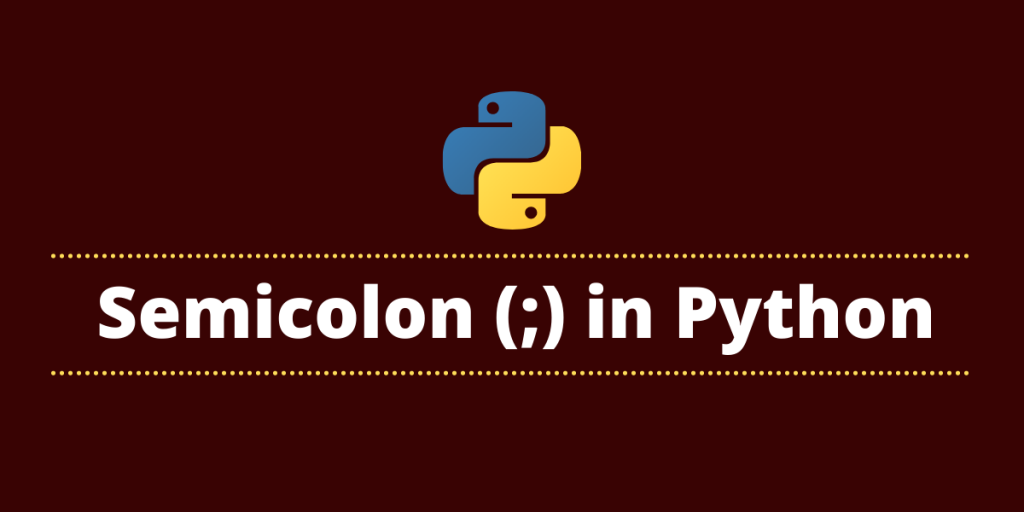# Semicolon in PythonLet’s talk about the use of the semicolon in Python. The general meaning of semicolon(;) in various programming languages is to put an end to or discontinue the current statement.

In programming languages like C, C++, and Java, using a semicolon is necessary to terminate the line of code. However, that is not the case with Python. So does using a semicolon make any difference in Python programming? Let’s find out.

## Why are semicolons allowed in Python?

Python does not require semi-colons to terminate statements. Semicolons can be used to delimit statements if you wish to put multiple statements on the same line.

semicolon in Python denotes separation, rather than termination. It allows you to write multiple statements on the same line. This syntax also makes it legal to put a semicolon at the end of a single statement. So, it’s actually two statements where the second one is empty.

## How to print a semicolon in Python?

Let’s see what happens when we try to print a semicolon as a regular string in Python

```>>> print(";")
```

Output:

```;
```

It treats the semicolon no differently and prints it out.

## Split Statements with Semicolons

Now, let’s see how we can split statements in Python with the use of semicolons. In this case, we’ll try to have more than 2 statements on the same line with the use of a semicolon.

Syntax:

```statement1; statement2
```

For Example:

Here are the three statements in Python without semicolons

```>>> print('Hi')
>>> print('Hello')
>>> print('Hola!')
```

Now let’s use the same three statement with semicolons

```print('Hi'); print('Hello'); print('Hola!')
```

Output:

```Hi
Hello
Hola!
```

As you can see, Python executes the three statements individually after we split them with semicolons. Without the use of that, the interpreter would give us an err.r

## Using Semicolons with Loops in Python

In loops like the ‘For loop‘, a semicolon can be used if the whole statement starts with a loop and you use a semicolon to form a coherent statement like the body of the loop.

Example:

```for i in range (4): print ('Hi') ; print('Hello')
```

Output:

```Hi
Hello
Hi
Hello
Hi
Hello
Hi
Hello
```

Python will throw an error if you use semicolon to seperate a normal expression from a block statement i.e loop.

Example:

```print('Hi') ; for i in range (4): print ('Hello')
```

Output:

```Invalid Syntax
```

## Conclusion

This brings us to the end of this brief module on the use of a semicolon in Python. Let’s summarize the tutorial with two pointers:

• A semicolon in Python is mostly used to separate multiple statements written on a single line.
• Semicolon is used to write minor statement and reserve a bit of space – like name = Marie; age = 23; print(name, age)

Use of semicolons is very “non-pythonic” and is best avoided unless you absolutely must use it.